Moments Flashcards Preview

Physics > Moments > Flashcards

Flashcards in Moments Deck (40):
1

2

3

4

what happened when Cici (more mass) and Tess (less mass) stand on different ends of a balance?Why?5

Explain why the see-saw became balanced with Tess and Nikita in this position relative to Cici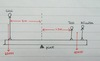6

7

8

9

10

11

which direction will the wheel turn?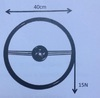12

describe two ways she could increase the moment on the wheel13

how do you find the centre of mass of an object?

suspend it from two different points (see figure)14

what is the method for finding the centre of mass of a piece of flat card?

suspend the flat card from a pin and let it swing freelywhen you do this, the centre of mass of the card is directly below the pinyou can use a 'plumbline' to draw a veritcal line on the card from the pin downwardsnow repeat this procedure with the card suspended from a different point to give another linethe centre of mass of the card is where the two lines cross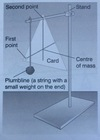15

What are A and B?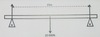16

A unifrom bridge has a weight of 20,000N. Two supports are placed near the ends, 7.5m from the centre of mass of the bridge. State the value of the support forces at A and B17

A car with a weight of 10,000N now parks at the centre of the bridge. State the extra force exerted at A and B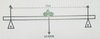18

A similar car drives from A to B at a constant speed. Describe the changes in the support forces acting on A and B, stating values of significant positions of the car along the bridge. You can state the total forces (which include the weight of the bridge), or extra forces (ignoring the weight of the bridge)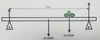19

how do you calculate the mass of ruler?

Wm x d1 = Wr x d2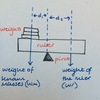20

Explain why Fb increases as the car approaches it21

Calulate the force of F22

Calculate the distance of d23

Calculate the force of F (assume cm is m instead)24

Explain why the ruler is equilibrium. Remember to refer to moments."A student investigated equilibrium by using the equiptment in the diagram. The 2N wieght is 60cm from the pivot and the Newton meter is 10.5cm from the pivot."25

Show that the upward force is about 10N"A student investigated equilibrium by using the equiptment in the diagram. The 2N wieght is 60cm from the pivot and the Newton meter is 10.5cm from the pivot."26

The student moves the 2N wieght further away from the pivot while the Newton meter remains in the same position. What happens to F, the value on the Newton meter?"A student investigated equilibrium by using the equiptment in the diagram. The 2N wieght is 60cm from the pivot and the Newton meter is 10.5cm from the pivot."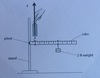27

Explain why F increases as the student moves the 2N weight further away from the pivot while the Newton meter remains in the same positionRefer to variables thar are changing and variables that do not change when the 2N weight is moved"A student investigated equilibrium by using the equiptment in the diagram. The 2N wieght is 60cm from the pivot and the Newton meter is 10.5cm from the pivot."28

A 900N car is parked on a uniform bridge as shown in the diagram. The bridge wieghs 11,000N and is 30m between A and B. The car is 10m from A.Add arrows and suitable lables (like FA) to represent the force at A, the force at B, the weight of the car, the weight of the bridge, the distance to the weight of the bridge and the distance to the weight of the car29

state which force will be greater; the upward force at A or the upward of force at B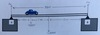30

As the bridge and the car is in equilibrium the resultant force on the system is equal to zeroUse this information (Nothing to do with moments yet) to write an equation linking the weight of the car, the weight of the bridge FA and FB31

32

Take moments about A to calculate the upward force at B33

The man in the diagram is using a device to hold a 200N bucket of water levelExplain in the system is in equilibrium or not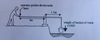34

The man in the diagram is using a device to hold a 200N bucket of water levelJohn says: "I think the man will have to exert more than 100N to keep the system in equilibrium."Evaluate his comment35

What will happen to the upward force at A and B as the car moves further away from A?36

37

38

39

40